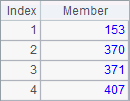# 循环流程

## for 循环

 A B C 1 =demo.query("select * from EMPLOYEE") [] 2 for =A1(A2) 3 if B2.STATE=="Texas" 4 >B1=B1|B2 5 if B1.len()==10 6 break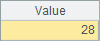## for A

 A B 1 =demo.query("select * from EMPLOYEE") 0 2 =A1.select(STATE=="Texas") 3 for A2 =age(A3.BIRTHDAY) 4 >B1=max(B1,B3)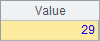A 1 =demo.query("select * from EMPLOYEE") 2 =A1.select(STATE=="Texas") 3 =A2.max(age(BIRTHDAY))

A3中的结果和上面的例子是相同的：## for n

n遍，主格的值依次为当前循环计数。

 A B C 1 1000 800 0.1 2 for 10 >A1=A1/2 >C1=C1*2 3 if B1>A1 =B1 4 >B1=A1 5 >A1=C3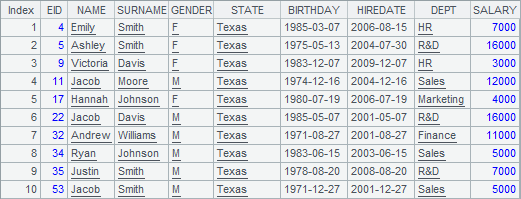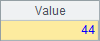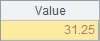## for a, b, s

ab执行循环，每次步进为s，即循环数列to(a,b).step(s)中的各个成员，s缺省时计为1

 A B 1 for 1,100,2 >A2=A2+A1 2

A2中计算100以内所有奇数的和，结果如下：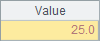A B C D 1 [] 2 for 100,999 =A2\100 =(A2\10)%10 =A2%10 3 =power(B2,3) =power(C2,3) =power(D2,3) 4 if B3+C3+D3==A2 >A1=A1|A2

A2的循环代码中，只循环所有的3位数，程序执行后，可以在A1中看到计算结果：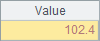## for x

x为真时循环，主格的值为x计算值。

 A B C 1 =demo.query("select * from EMPLOYEE") [] 2 for B1.len()<10 =A1(#A2) 3 if B2.STATE=="Texas" 4 >B1=B1|B2

for x循环的主格中，格值为每次计算循环条件的结果，为true或者false。这时不能用主格中的值来获得循环次数，因此在B2中获取员工记录时，使用了#C来获得当前的循环次数，#C中，C为循环体的主格。代码执行后，结果与中相同。

## 循环的嵌套

 A B C D 1 [] 2 for 2,1000 for A1 if A2%B2==0 next A2 3 >A1=A1|A2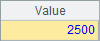A B C D 1 [] 2 for 100 if 5*A2>100 break 3 for 100-A2 =100-A2-B3 =5*A2+3*B3+C3/3 4 if D3>100 next A2 5 else if D3<100 next 6 >A1=A1|[[A2,B3,C3]]